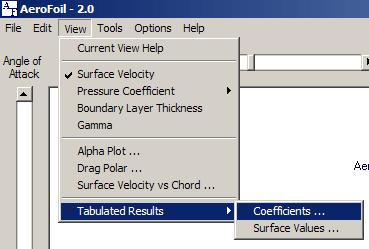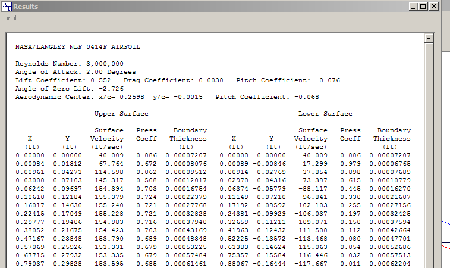## Tabulated dataTwo different displays of tabulated data are available; these are coefficients and surface values.

The coefficient display shows the following information:

 Angle of Zero Lift Aerodynamic Center Pitch Coefficient about the aerodynamic center Reynolds Number Angle of Attack Lift Coefficient Pitch Coefficient If viscous effects are included: Drag Coefficient Chord location, in terms of x/c, of transition points from: Laminar flow to turbulent flow Turbulent flow to separation lift-to-drag ratioThe surface value display shows the following:

• The X and Y coodinate points which define the airfoil,
• The surface velocity, pressure coefficient,
• and the boundary layer thickness (if viscous effects are included) at each point.

These values are a linear interpolation at the selected angle of attack. A negative value of surface velocity represents flow which is below the stagnation point and is passing below the airfoil.